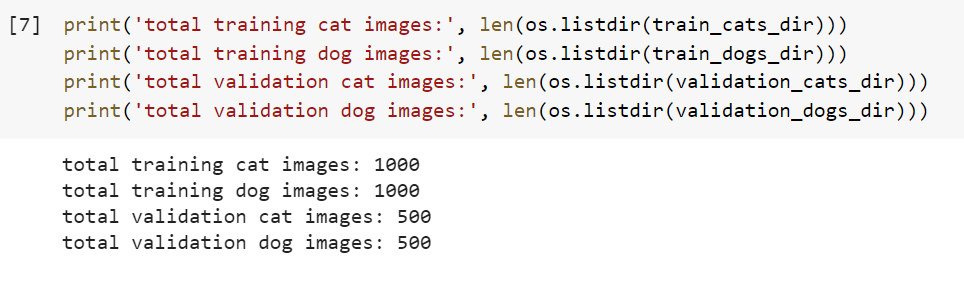Problem: Transfer learning train a model on less data (<1000 images) and still getting good results (>80% accuracy) to demonstrate the use of transfer learning. You are free to pick a model of your choice and a dataset of your choice. (just don’t pick dataset which is already used in initial training)

In this page I am going to solve the above problem, I will implement the binary-class image classification using the VGG-16 Deep Convolutional Network used as a Transfer Learning framework where the VGGNet comes pre-trained on the ImageNet dataset. For the experiment, we will use the Kaggle dogs-vs-cats dataset and classify the image objects into 2 classes. The classification accuracies of the VGG-16 model will be visualized using the confusion matrices.

## What is Transfer Learning?

Transfer learning is a research problem in the field of machine learning. It stores the knowledge gained while solving one problem and applies it to a different but related problem. In deep learning, transfer learning is a technique whereby a neural network model is first trained on a problem similar to the problem that is being solved. Transfer learning has the advantage of decreasing the training time for a learning model and can result in lower generalization error.

## VGGNet – The Deep Convolutional Network

VGGNet is a Deep Convolutional Neural Network that was proposed by Karen Simonyan and Andrew Zisserman of the University of Oxford in their research work ‘Very Deep Convolutional Neural Networks for Large-Scale Image Recognition’. The name of this model was inspired by the name of their research group ‘Visual Geometry Group (VGG)’. As this convolutional neural network has 16 layers in its architecture, it was named VGG-16. This model was proposed to reduce the number of parameters in a convolutional neural network with improved training time.

The biggest advantage of this network is that we can load a pre-trained version of the network trained on more than a million images from the ImageNet database. A pre-trained network can classify images into thousands of object categories. Due to this advantage, I am going to apply this model to the Kaggle dogs-vs-cats dataset image dataset that has 2 object categories.

## The Dataset

To complete this task, I shall be using the Kaggle dogs-vs-cats dataset that is a publically available image data set provided by the Kaggle. It consists of 25000 colour images in 2 classes as a training dataset. I work on only the train dataset so I am not going to discuss the test dataset and here I am talking about the raw dataset which is actually the training dataset as discussed above. I will slice the first 1000 (raw_dataset[:1000]) images from the original raw dataset, out of which 503 are dog and 497 are the cat and then split it into validating as 100 and 900 as train. To test our model I have sliced 200 datasets from the original raw dataset[10000:10200].

## Importing libraries

```import numpy as np
import pandas as pd
import os
from keras.preprocessing.image import ImageDataGenerator, load_img
from keras.utils import to_categorical
from sklearn.model_selection import train_test_split
import matplotlib.pyplot as plt
import random
from keras import layers
from keras.layers import Conv2D, MaxPooling2D, Dropout, Flatten, Dense, Activation,GlobalMaxPooling2D
from keras import applications
from keras.preprocessing.image import ImageDataGenerator
from keras import optimizers
from keras.applications import VGG16
from keras.models import Model
import seaborn as sns
from sklearn.metrics import accuracy_score
from sklearn.metrics import confusion_matrix
```

## Importing the Dataset

```!wget --no-check-certificate \
-O /tmp/cats_and_dogs_filtered.zip

import os
import zipfile

local_zip = '/tmp/cats_and_dogs_filtered.zip'
zip_ref = zipfile.ZipFile(local_zip, 'r')
zip_ref.extractall('/tmp')
zip_ref.close()

base_dir = '/tmp/cats_and_dogs_filtered'
train_dir = os.path.join(base_dir, 'train')
validation_dir = os.path.join(base_dir, 'validation')

# Directory with our training cat pictures
train_cats_dir = os.path.join(train_dir, 'cats')

# Directory with our training dog pictures
train_dogs_dir = os.path.join(train_dir, 'dogs')

# Directory with our validation cat pictures
validation_cats_dir = os.path.join(validation_dir, 'cats')

# Directory with our validation dog pictures
validation_dogs_dir = os.path.join(validation_dir, 'dogs')
```

Let’s find out the total number of cat and dog images in the `train` and `validation` directories:

```train_cat_fnames = os.listdir(train_cats_dir)
print(train_cat_fnames[:10])

train_dog_fnames = os.listdir(train_dogs_dir)
train_dog_fnames.sort()
print(train_dog_fnames[:10])
```

Let’s find out the total number of cat and dog images in the train and validation directories:

```print('total training cat images:', len(os.listdir(train_cats_dir)))
print('total training dog images:', len(os.listdir(train_dogs_dir)))
print('total validation cat images:', len(os.listdir(validation_cats_dir)))
print('total validation dog images:', len(os.listdir(validation_dogs_dir)))
``````IMAGE_FOLDER_PATH="../working/train"
FILE_NAMES=os.listdir(IMAGE_FOLDER_PATH)
WIDTH=150
HEIGHT=150

targets=list()
full_paths=list()
for file_name in FILE_NAMES:
target=file_name.split(".")
full_path=os.path.join(IMAGE_FOLDER_PATH, file_name)
full_paths.append(full_path)
targets.append(target)

raw_dataset=pd.DataFrame()
raw_dataset['image_path']=full_paths
raw_dataset['target']=targets

```